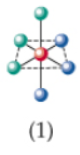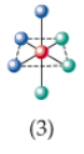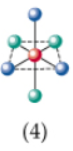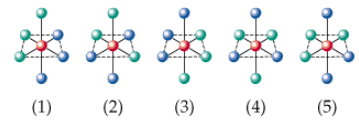# Problem: There are two geometric isomers of octahedral complexes of the type MA3X3 (Figure 1), where M is a metal and A and X are monodentate ligands.Of the complexes shown here, which are identical to (1) and which are the geometric isomers of (1)? Complex 2, Complex 3, Complex 4, Complex 5.

###### FREE Expert Solution

We are asked which are identical to (1) and which are the geometric isomers of (1).

Rotating the structures will show us that it is identical to (1):Rotating (3) about the vertical axis by 180° will make it look like (1):Rotating (3) about the vertical axis by 90° will make it look like (1):91% (12 ratings)###### Problem Details

There are two geometric isomers of octahedral complexes of the type MA3X3 (Figure 1), where M is a metal and A and X are monodentate ligands.

Of the complexes shown here, which are identical to (1) and which are the geometric isomers of (1)?

Complex 2, Complex 3, Complex 4, Complex 5.Frequently Asked Questions

What scientific concept do you need to know in order to solve this problem?

Our tutors have indicated that to solve this problem you will need to apply the Coordination Complexes concept. You can view video lessons to learn Coordination Complexes. Or if you need more Coordination Complexes practice, you can also practice Coordination Complexes practice problems.